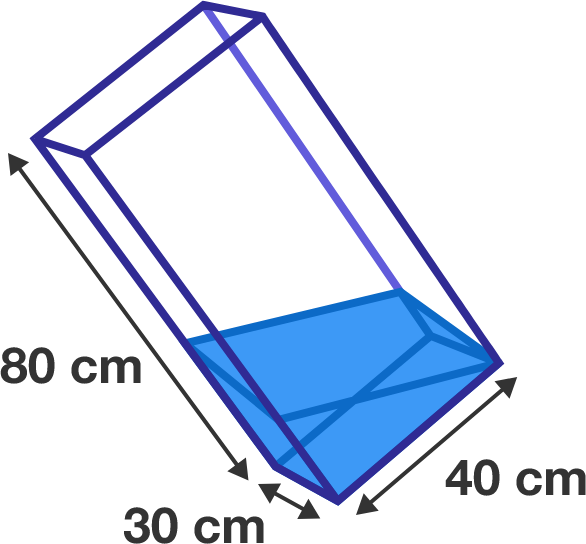# Cuboid water tank

Geometry Level 3A water tank is in the shape of a cuboid with a height of $80\text{ cm}$ and a base that measures $30\text{ cm} \times 40\text{ cm}.$

It is tilted so that its space diagonal (the longest diagonal between two opposing vertices) becomes vertical.

Next, water is poured into the tank up to the highest point of the tilted base, as shown in the diagram to the right.

How much water (in cubic centimeters) has been poured in?

×